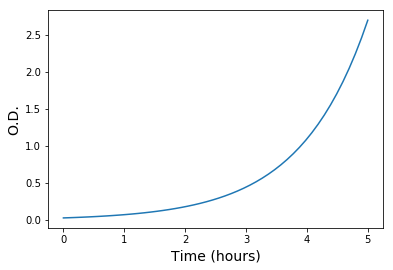### Exponential Growth

Unicellular organisms like Escherichia coli reproduce by something called as binary fission. It is essentially the division of one bacterial cell into two. When they are grown in laboratory in nutrient medium they tend to divide exponentially until the nutrients in the medium get consumed by them. Exponential growth phase can be represented as a mathematical equation.
Xt= X0 exp(mu  * t)
where, X0 = the amount of cells at initial time (to)
Xt = the amount of cells at time (t)
mu = specific growth rate (per unit time)
This can be modelled easily in Python.
We first make a function to calculate the amount cells at any time with initial biomas and growth rate as input.
In :
```import numpy as np
import matplotlib.pyplot as plt
%matplotlib inline

def biomass_t(biomass_0,growth_rate,time):
# calculates final biomass
import math
return biomass_0 * math.exp(growth_rate * time)

```

### Example:¶

Let's say the specific growth rate of the bacteria is 0.9 per hour and initial optical density of culure is 0.03. The following code will predict the O.D. for 5 hours. We generate an array of values from 0 to 5 which represent the time. For each of these time points we calculate the biomass. Here we have set the initial biomass as 0.03 and growth rate as 0.9 per hour. The biomass units can be in gram dry weight or optical density (OD). The values used here are in terms of OD.
In :
```x = np.linspace(0,5)
y = np.array([biomass_t(biomass_0=0.03, growth_rate=0.9,time=k) for k in x])
plt.plot(x,y)
plt.xlabel('Time (hours)', size = 14)
plt.ylabel('O.D.', size = 14)
```
Out:
`<matplotlib.text.Text at 0x17ef2753b70>`In [ ]:
```
```